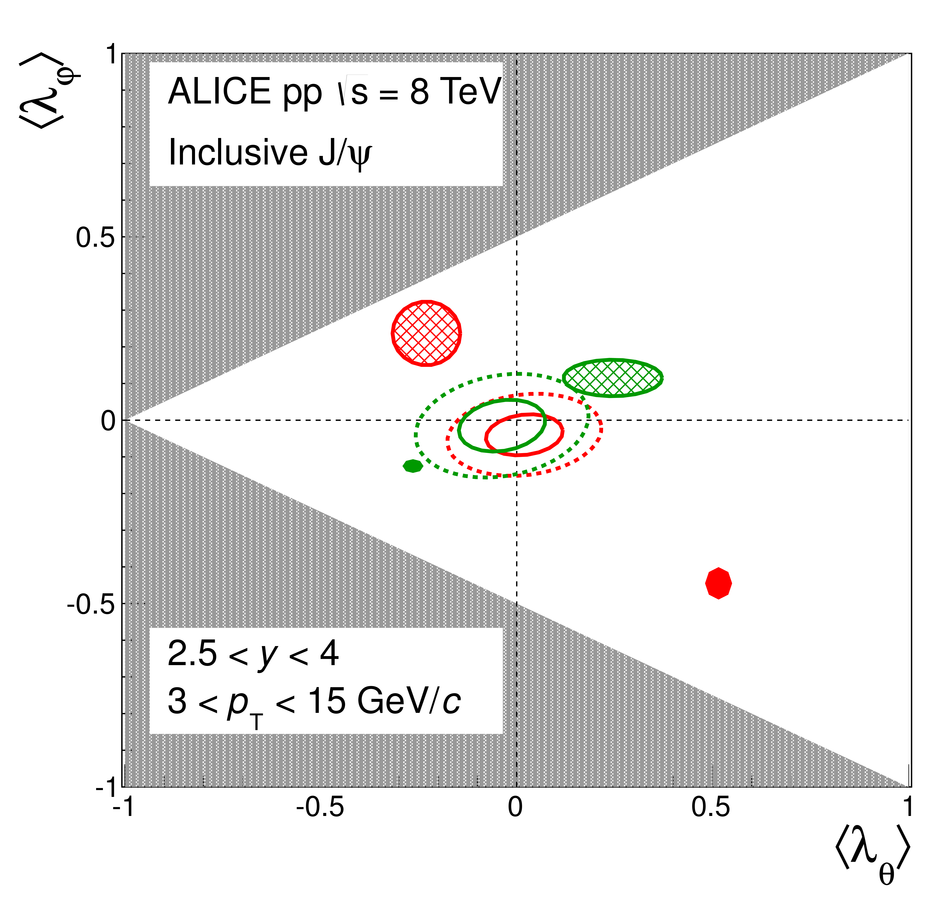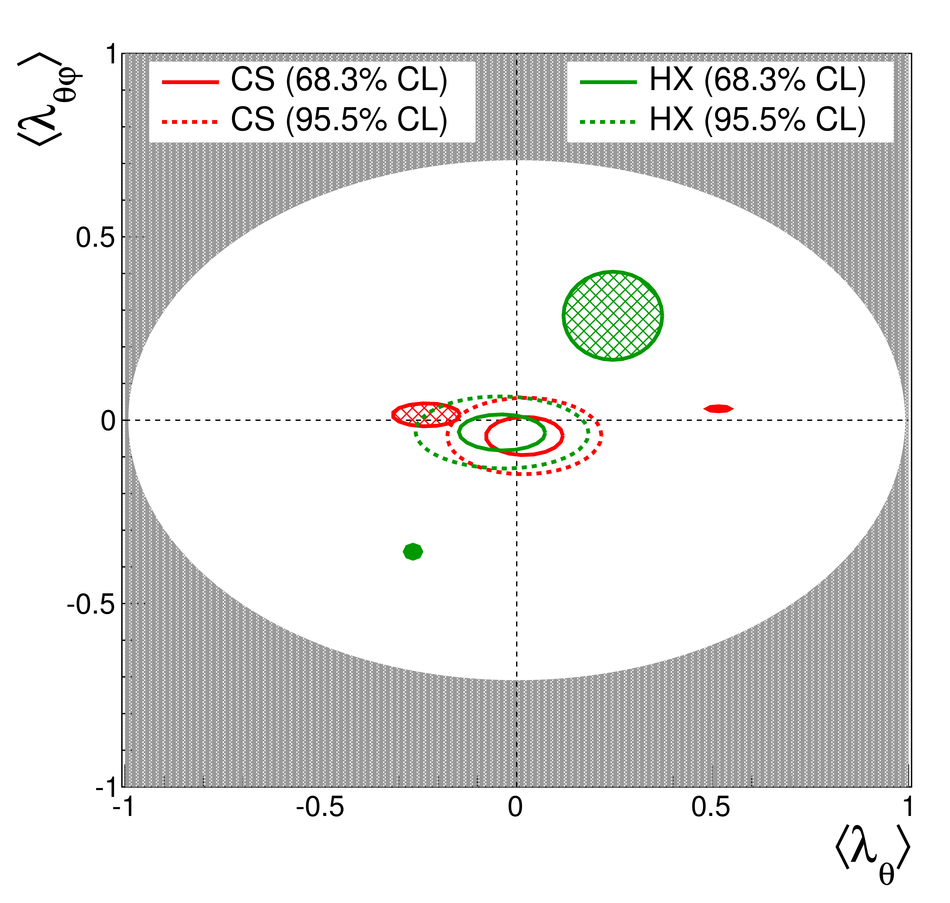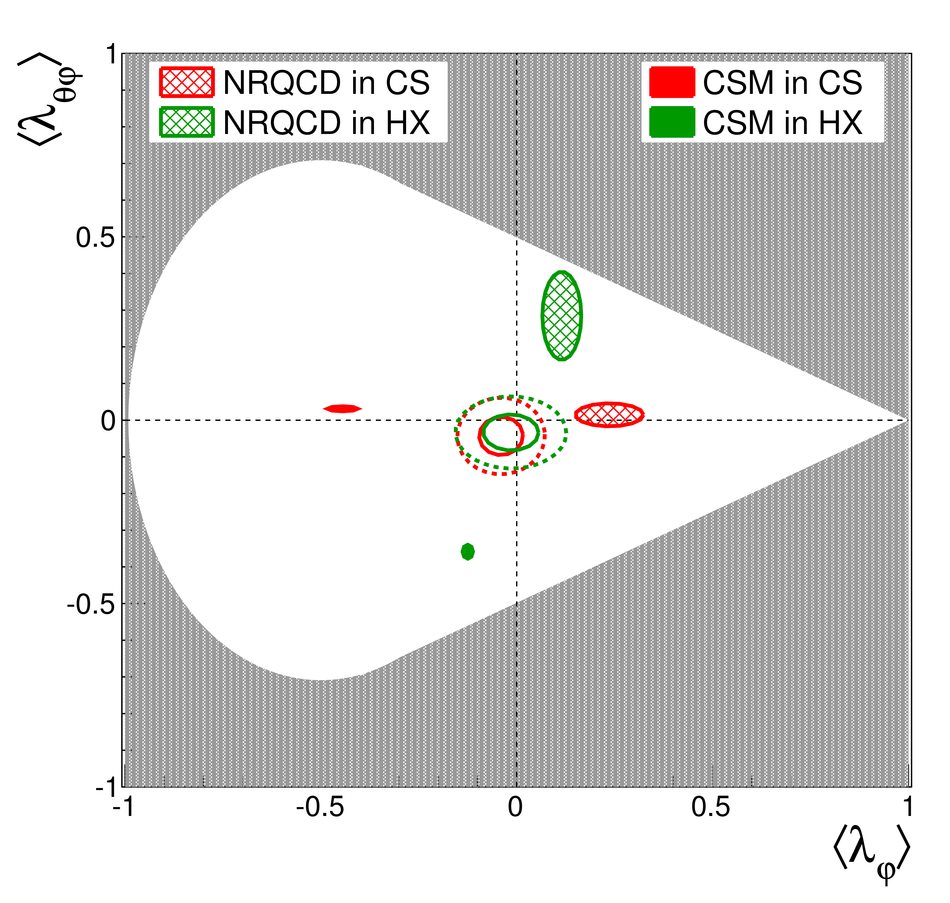Figure 6

 Average $p_{\rm T}$-integrated (in rapidity range $2.5 < y < 4.0$) inclusive J/$\psi$ polarization parameters $\langle\lambda_\theta\rangle$, $\langle\lambda_\varphi\rangle$ and $\langle\lambda_{\theta\varphi}\rangle$ in allowed 2-D regions (white areas) for $3 < p_{\rm T} < 15$~\GeVc. Full (dashed) ellipses show 1-$\sigma$ (2-$\sigma$) contours in Collins-Sopper (CS, red) and helicity (HX, green) frames. Model predictions~ are represented by filled contours, full filled for the CSM and shaded filled for the NRQCD model, in green for the HX frame and in red for the CS frame.